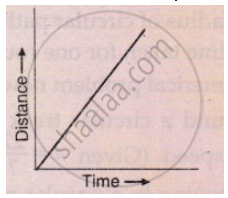Share

# What Conclusion Can You Draw About the Speed of a Body from the Following Distance-time Graph ? - CBSE Class 9 - Science

ConceptGraphical Representation of Motion - Distance-time Graphs

#### Question

What conclusion can you draw about the speed of a body from the following distance-time graph ?#### Solution

The body has a uniform speed  because the body covers equal distance in equal interval of time.

Is there an error in this question or solution?

#### APPEARS IN

Lakhmir Singh Solution for Science for Class 9 Part 1 Physics (2016 to Current)
Chapter 1: Motion
Very Short Answers | Q: 11 | Page no. 40

#### Video TutorialsVIEW ALL 

Solution What Conclusion Can You Draw About the Speed of a Body from the Following Distance-time Graph ? Concept: Graphical Representation of Motion - Distance-time Graphs.
S